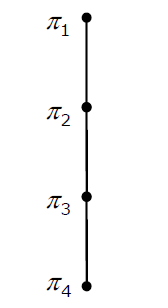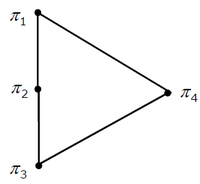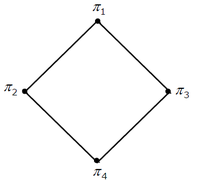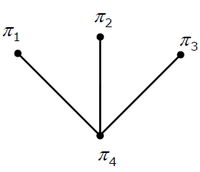# Set Theory & Algebra

 Question 1
A binary operationon a set of integers is defined as xy = x2 + y2. Which one of the following statements is TRUE about?Commutative but not associativeBoth commutative and associativeAssociative but not commutativeNeither commutative nor associative

Question 1-Explanation:
Associativity: A binary operation ∗ on a set S is said to be associative if it satisfies the associative law: a ∗ (b ∗c) = (a ∗b) ∗c for all a, b, c ∈S. Commutativity: A binary operation ∗ on a set S is said to be commutative if it satisfies the condition: a ∗b=b ∗a for all a, b, ∈S. In this case, the order in which elements are combined does not matter. Solution: Here a binary operation on a set of integers is defined as x⊕ y = x2 + y2. for Commutativity: x ⊕y= y ⊕x. LHS=> x ⊕y= x^2+ y^2 RHS=> y ⊕x= y^2+x^2 LHS = RHS. hence commutative. for Associativity: x ⊕ (y ⊕ z) =(x ⊕ y) ⊕ z LHS=> x ⊕ (y⊕ z) = x ⊕ ( y^2+z^2)= x^2+(y^2+z^2)^2 RHS=> (x ⊕y) ⊕z= ( x^2+y^2) ⊕z=(x^2+y^2)^2+z^2 So, LHS ≠ RHS, hence not associative. Reference: http://faculty.atu.edu/mfinan/4033/absalg3.pdf This solution is contributed by Nitika Bansal Another Solution :commutative as xy is always same as yx.is not associative as (xy)z is (x^2 + y^2)^2 + z^2, but x(yz) is x^2 + (y^2 + z^2)^2.
 Question 2
Consider the set S = {1, ω, ω2}, where ω and w2 are cube roots of unity. If * denotes the multiplication operation, the structure (S, *) formsA groupA ringAn integral domainA field

Question 2-Explanation:
A group is a set of elements together with an operation that combines any two of its elements to form a third element also in the set while satisfying four conditions called the group axioms, namely closureassociativityidentity and invertibility. (Source: http://en.wikipedia.org/wiki/Group_(mathematics) (S, *)  is a group with identity as 1
 Question 3
Which one of the following in NOT necessarily a property of a Group?CommutativityAssociativityExistence of inverse for every elementExistence of identity

Question 3-Explanation:
A group is a set, G, together with an operation • (called the group law of G) that combines any two elements a and b to form another element, denoted a • b or ab. To qualify as a group, the set and operation, (G, •), must satisfy four requirements known as the group axioms: Closure For all a, b in G, the result of the operation, a • b, is also in G.b Associativity For all a, b and c in G, (a • b) • c = a • (b • c). Identity element There exists an element e in G, such that for every element a in G, the equation e • a = a • e = a holds. Such an element is unique (see below), and thus one speaks of the identity element. Inverse element For each a in G, there exists an element b in G such that a • b = b • a = e, where e is the identity element. The result of an operation may depend on the order of the operands. In other words, the result of combining element a with element b need not yield the same result as combining element b with element a; the equation a • b = b • a may not always be true. This equation always holds in the group of integers under addition, because a + b = b + a for any two integers (commutativity of addition). Groups for which the commutativity equation a • b = b • a always holds are called abelian groups (in honor of Niels Abel) Source: http://en.wikipedia.org/wiki/Group_(mathematics)
 Question 4
Consider the binary relation R = {(x, y), (x, z), (z, x), (z, y)} on the set {x, y, z}. Which one of the following is TRUE?R is symmetric but NOT antisymmetricR is NOT symmetric but antisymmetricR is both symmetric and antisymmetricR is neither symmetric nor antisymmetric

Question 4-Explanation:
R is not symmetric as (x, y) is present, but (y, x) is not present in R. R is also not antisymmetric as both (x, z) and (z, x) are present in R.
 Question 5
For the composition table of a cyclic group shown below
 * a b c d a a b c d b b a d c c c d b a d d c a b
Which one of the following choices is correct?a, b are generatorsb, c are generatorsc, d are generatorsd, a are generators

Question 5-Explanation:
```Check for all:-
a1 = a ,
a2 = a * a = a
a3 = a2 * a = a * a = a
a is not the generator since we are not able to express
other members of the group in powers of a

Check for c -
c1 = c
c2 = c * c = b
c3 = c2 * c = b * c = d
c4 = c2 * c2 = b * b = a
We are able to generate all the members of the group from c ,
Hence c is the generator

Similarly check for d
```
 Question 6Qc U RcP U Qc U RcPc U Qc U RcU

Question 6-Explanation:

The given set theory expression can be converted into an equivalent boolean algebra expression as follows:

=p.q.r + p'.q.r + q'+r'

=qr(p+p')+q'+r'

=qr+q'+r'

=(q+q') . (r+q') +r'

=r+r'+q'

=1+q'

=1

=U

Option (D) is correct

 Question 7
Let S be a set of nelements. The number of ordered pairs in the largest and the smallest equivalence relations on S are:n and nn2 and nn2 and 0n and 1

Question 7-Explanation:
```Consider an example set, S = (1,2,3)

Equivalence property follows, reflexive, symmetric
and transitive

Largest ordered set are s x s =
{ (1,1) (1,2) (1,3) (2,1) (2,2) (2,3) (3,1) (3,2)
(3,3) } which are 9 which equal to 3^2 = n^2

Smallest ordered set are { (1,1) (2,2) ( 3,3)}
which are 3 and equals to n. number of elements.```
 Question 8
How many different non-isomorphic Abelian groups of order 4 are there2345

Question 8-Explanation:
```2 can be written as 2 power 2.
Number of partitioning of 2 = no. of non isomorphic
abelian groups
2 can be partitioned as {(2),(1,1)}```
 Question 9

Consider the set S = (A, B, C, D}. Consider the following 4 partitions π1, π2, π3, π4 on S : π1 = {}, π2 = {}, π3 = {}, π4 = {}. Let P be the partial order on the set of partitions S' = {π1, π2, π3, π4} defined as follows : πi P πj if and only if πi refines πj. The poset diagram for (S', P ) is :Question 9-Explanation:

A partition is said to refine another partition if it splits the sets in the second partition to a larger number of sets.
Corresponding Hasse diagram is Option C.

 Question 10ABCD

Question 10-Explanation:
To be basis of subspace x, 2 conditions are to be fulfilled 1) They must span x 2) The vectors have to be linearly independent 1)the general solution of x1+x2+x3=0 is [-x2-x3 , x2 , x3]^T (Transpose) Which gives two linearly independent solutions by assuming x2 = 1 and x3 = 0 and next x3 = 1 and x2 = 0 gives [-1,1,0]^T and [-1,0,1]^T respectively. Since both of these can be generated by linear combinations of [1,-1,0]^T & [-1,0,1]^T given in question, it span x. 2) Above set of column vector is linearly independent because one cannot be obtained from another by scalar multiplication (second method rank is 2..that is why linearly independent)
There are 122 questions to complete.
• Last Updated : 21 Jan, 2014